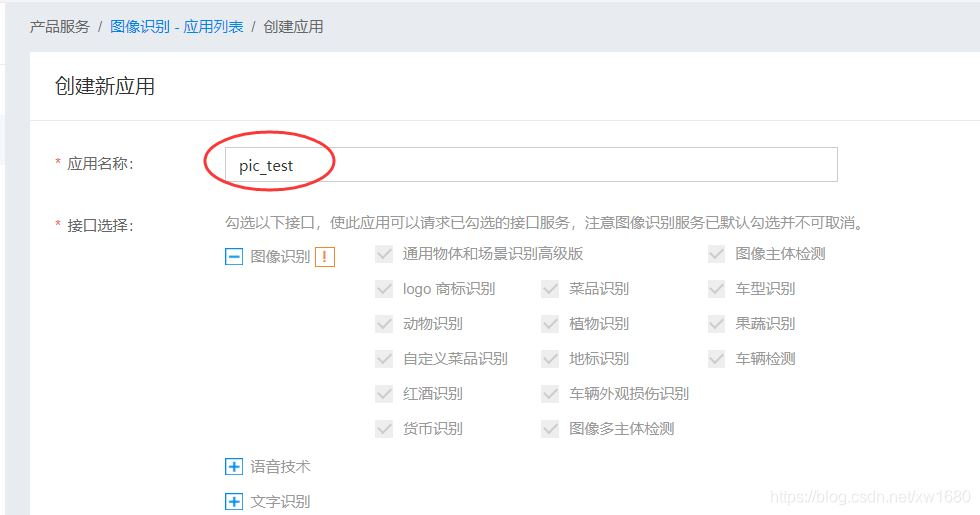# Python基於百度AI實現抓取表情包

## 一、百度 AI 開放平臺的 Key 申請方法## 二、抓取貼吧表情包

Network 抓包看下返回的數據是否和 Element 一致，即是否包含想要的數據，而不是通過 JS 黑魔法進行加載的。復制下第一個圖的圖片鏈接，到 Network 選項卡裡的 Response 裡查找一下。```# 抓取百度貼吧某個帖子裡的所有圖片
import requests
import time
import queue
from bs4 import BeautifulSoup
import chardet
import os

tiezi_url = "https://tieba.baidu.com/p/5522091060"
'Host': 'tieba.baidu.com',
'User-Agent': 'User-Agent: Mozilla/5.0 (Windows NT 10.0; Win64; x64) AppleWebKit/537.36 (KH'
'TML, like Gecko) Chrome/90.0.4430.212 Safari/537.36',
}
pic_save_dir = 'tiezi_pic/'
if not os.path.exists(pic_save_dir):  # 判斷文件夾是否存在，不存在就創建
os.makedirs(pic_save_dir)

pic_urls_file = 'tiezi_pic_urls.txt'

# 獲得頁數
def get_page_count():
try:
if resp is not None:
resp.encoding = chardet.detect(resp.content)['encoding']
html = resp.text
soup = BeautifulSoup(html, 'lxml')
a_s = soup.find("ul", attrs={'class': 'l_posts_num'}).findAll("a")
for a in a_s:
if a.get_text() == '尾頁':
return a['href'].split('=')
except Exception as e:
print(str(e))

# 下載線程
def __init__(self, t_name, func):
self.func = func

def run(self):
self.func()

# 獲得每頁裡的所有圖片URL
def get_pics(count):
params = {
'pn': count,
'ajax': '1',
't': int(time.time())
}
try:
if resp is not None:
resp.encoding = chardet.detect(resp.content)['encoding']
html = resp.text
soup = BeautifulSoup(html, 'lxml')
imgs = soup.findAll('img', attrs={'class': 'BDE_Image'})
for img in imgs:
print(img['src'])
with open(pic_urls_file, 'a') as fout:
fout.write(img['src'])
fout.write('\n')
return None
except Exception:
pass

# 下載線程調用的方法
def down_pics():

# 下載調用的方法
try:
if resp.status_code == 200:
print("下載圖片:" + img_url)
pic_name = img_url.split("/")[-1][0:-1]
with open(pic_save_dir + pic_name, "wb+") as f:
f.write(resp.content)

except Exception as e:
print(e)

if __name__ == '__main__':
print("檢索判斷鏈接文件是否存在：")
if not os.path.exists(pic_urls_file):
print("不存在，開始解析帖子...")
page_count = get_page_count()
if page_count is not None:
for page in range(1, int(page_count) + 1):
get_pics(page)
print("鏈接已解析完畢！")
else:
print("存在")
print("開始下載圖片~~~~")
fo = open(pic_urls_file, "r")

for pic in pic_list:
for i in range(0, len(pic_list)):
t = PicSpider(t_name='線程' + str(i), func=down_pics)
t.daemon = True
t.start()
t.join()
print("圖片下載完畢")
```## 三、使用 Baidu-aip

```pip install baidu-aip
```

```from aip import AipOcr

# 新建一個AipOcr對象
config = {
'appId': '填寫自己的appId',
'apiKey': '填寫自己的apiKey',
'secretKey': '填寫自己的secretKey'
}
client = AipOcr(**config)

# 識別圖片裡的文字
def img_to_str(image_path):
# 讀取圖片
with open(image_path, 'rb') as fp:

# 調用通用文字識別, 圖片參數為本地圖片
result = client.basicGeneral(image)
# 返回拼接結果
if 'words_result' in result:
return '\n'.join([w['words'] for w in result['words_result']])

if __name__ == '__main__':
``````{'words_result': [{'words': 'o。o'}, {'words': '6226-16:59'}, {'words': '絕望jpg'}], 'log_id': 1393611954748129280, 'words_result_num': 3}
o。o
6226-16:59

```

```# 識別圖片文字，批量命名圖片文字

import os
from aip import AipOcr
import re
import datetime

# 新建一個AipOcr對象
config = {
'appId': '填寫自己的appId',
'apiKey': '填寫自己的apiKey',
'secretKey': '填寫自己的secretKey'
}
client = AipOcr(**config)

pic_dir = r"tiezi_pic/"

# 讀取圖片
def get_file_content(file_path):
with open(file_path, 'rb') as fp:

# 識別圖片裡的文字
def img_to_str(image_path):
image = get_file_content(image_path)
# 調用通用文字識別, 圖片參數為本地圖片
result = client.basicGeneral(image)
# 結果拼接返回
words_list = []
if 'words_result' in result:
if len(result['words_result']) > 0:
for w in result['words_result']:
words_list.append(w['words'])
file_name = get_longest_str(words_list)
print(file_name)
file_dir_name = pic_dir + str(file_name).replace("/", "") + '.jpg'
if os.path.exists(file_dir_name):  # 處理文件重名問題
sec = datetime.datetime.now().microsecond  # 獲取當前毫秒時值
file_dir_name = pic_dir + str(file_name).replace("/", "") + str(sec) + '.jpg'
try:
os.rename(image_path, file_dir_name)
except Exception:
print(" 重命名失敗：", image_path, " => ", file_name)

# 獲取字符串列表中最長的字符串
def get_longest_str(str_list):
pat = re.compile(r'[\u4e00-\u9fa5A-Za-z]+')
str = max(str_list, key=hanzi_len)
result = pat.findall(str)
return ''.join(result)

def hanzi_len(item):
pat = re.compile(r'[\u4e00-\u9fa5]+')
sum = 0
for i in item:
if pat.search(i):
sum += 1
return sum

# 遍歷某個文件夾下所有圖片
def query_picture(dir_path):
pic_path_list = []
for filename in os.listdir(dir_path):
pic_path_list.append(dir_path + filename)
return pic_path_list

if __name__ == '__main__':
pic_list = query_picture(pic_dir)
if len(pic_list) > 0:
for i in pic_list:
img_to_str(i)
```